Please wait a minute...
 Chin. Phys. B, 2020, Vol. 29(11): 110307    DOI: 10.1088/1674-1056/aba614
 GENERAL Prev   Next

# Protecting the entanglement of two-qubit over quantum channels with memory via weak measurement and quantum measurement reversal

Mei-Jiao Wang(王美姣)1, Yun-Jie Xia(夏云杰)2,3, Yang Yang(杨阳)1, Liao-Zhen Cao(曹连振)1, Qin-Wei Zhang(张钦伟)1, Ying-De Li(李英德)1, and Jia-Qiang Zhao(赵加强)1, †
1 Department of Physics and Optoelectronic Engineering, Weifang University, Weifang 261061, China
2 College of Physics and Engineering, Qufu Normal University, Qufu 273165, China
3 Shandong Provincial Key Laboratory of Laser Polarization and Information Technology, Qufu Normal University, Qufu 273165, China
 Abstract  Based on the quantum technique of the weak measurement and quantum measurement reversal (WMR), we propose a scheme to protect entanglement for an entangled two-qubit pure state from four typical quantum noise channels with memory, i.e., the amplitude damping channel, the phase damping channel, the bit flip channel, and the depolarizing channel. For a given initial state | ψ 〉 = a| 00 〉 + d| 11 〉, it is found that the WMR operation indeed helps to protect entanglement from the above four quantum channels with memory, and the protection effect of WMR scheme is better when the coefficient a is small. For the other initial state | ϕ 〉 = b| 01 〉 + c| 10 〉, the effect of the protection scheme is the same regardless of the coefficient b and the WMR operation can protect entanglement in the amplitude damping channel with memory. Moreover, the protection of entanglement in quantum noise channels without memory in contrast to the results of the channels with memory is more effective. For | ψ 〉 or | ϕ 〉, we also find that the memory parameters play a significant role in the suppression of entanglement sudden death and the initial entanglement can be drastically amplified. Another more important result is that the relationship between the concurrence, the memory parameter, the weak measurement strength, and quantum measurement reversal strength is found through calculation and discussion. It provides a strong basis for the system to maintain maximum entanglement in the nosie channel. Keywords:  quantum entanglement      weak measurement and quantum measurement reversal      quantum channel with memory      concurrence Received:  08 May 2020      Revised:  06 July 2020      Published:  03 November 2020 Fund: the Natural Science Foundation of Shandong Province, China (Grant No. ZR2017MF040). Corresponding Authors:  †Corresponding author. E-mail: zhaojiaqiang@wfu.edu.cn

#### Cite this article:

Mei-Jiao Wang(王美姣), Yun-Jie Xia(夏云杰), Yang Yang(杨阳), Liao-Zhen Cao(曹连振), Qin-Wei Zhang(张钦伟), Ying-De Li(李英德), and Jia-Qiang Zhao(赵加强) Protecting the entanglement of two-qubit over quantum channels with memory via weak measurement and quantum measurement reversal 2020 Chin. Phys. B 29 110307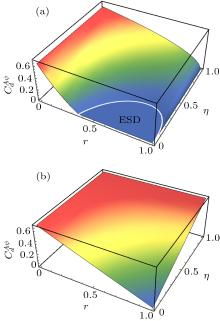Fig. 1.  In the AD channel with memory, the concurrence ${C}_{d}^{A\psi (\phi)}$ is the function of decoherent strength r and the memory parameter η for two initial entangled states | ψ 〉 = a|00〉 + d| 11 〉 and | ϕ 〉 = b| 01 〉 + c| 10 〉. We assume (a) | a | = 1 / 3, (b) | b | = 1 / 3.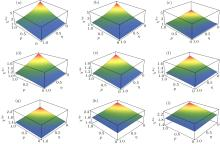Fig. 2.  In the AD channel with memory, χAψ is a function of the weak measurement strength p and the memory parameter η for the initially entangled states | ψ 〉 = a| 00 〉 + d| 11 〉. We assume r = 0.2, (a) $a=\sqrt{0.1}$; (b) a = 1 / 3; (c) $a=\sqrt{0.2}$; (d) $a=\sqrt{0.4}$; (e) $a=\sqrt{2}/2$; (f) $a=\sqrt{0.6}$; (g) $a=\sqrt{0.8}$; (h) $a=\sqrt{8}/3$; (i) $a=\sqrt{0.9}$.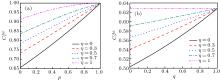Fig. 3.  In the AD channel with memory, the concurrence ${C}_{r}^{A\psi (\phi)}$ is a function of WM strength p (q) for the two initially entangled states | ψ 〉 = a| 00 〉 + d| 11 〉 and | ϕ 〉 = b| 01 〉 + c| 10 〉. We assume r = 0.2, (a) | a | = 1 / 3, (b) | b | = 1/3.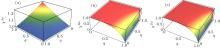Fig. 4.  In the quantum channels with memory, χA(B,D)ϕ is a function of the weak measurement strength p and the memory parameter η for the initially entangled state | ϕ 〉 = b| 01 〉 + c| 10 〉. We assume r = 0.2 and | b | = 1 / 3.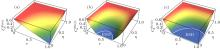Fig. 5.  In the quantum channels with memory, the concurrence ${C}_{d}^{P(B,D)\psi (\phi)}$ is a function of decoherent strength r and the memory parameter η for the two initial entangled states | ψ 〉 = a| 00 〉 + d| 11 〉 and | ϕ 〉 = b| 01 〉 + c| 10 〉. We assume | a | = 1 / 3 and | b | = 1 / 3. (a) PD channel; (b) BF channel; (c) depolarizing channel.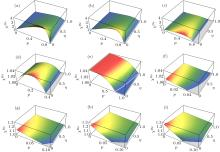Fig. 6.  In the BF channel with memory, ${\chi }^{B\psi }$ is a function of the weak measurement strength p and the memory parameter η for the initially entangled state | ψ 〉 = a| 00 〉 + d| 11 〉. We assume r = 0.2, (a) $a=\sqrt{0.1}$; (b) a = 1 / 3; (c) $a=\sqrt{0.2}$; (d) $a=\sqrt{0.4}$; (e) $a=\sqrt{2}/2$; (f) $a=\sqrt{0.6}$; (g) $a=\sqrt{0.8}$; (h) $a=\sqrt{8}/3$; (i) $a=\sqrt{0.9}$.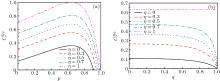Fig. 7.  In the BF channel with memory, the concurrence ${C}_{r}^{B\psi (\phi)}$ is a function of weak measurement strength p (q) for two initially entangled states | ψ 〉 = a| 00 〉 + d| 11 〉 and | ϕ 〉 = b| 01 〉 + c| 10 〉. We assume r = 0.2, (a) | a | = 1 / 3, (b) | b | = 1 / 3.
  Horodedecki R, Horodedecki P, Horodedecki M, Horodedecki K 2009 Rev. Mod. Phys. 81 865 DOI: 10.1103/RevModPhys.81.865  Zyczkowski K, Horodedecki P, Horodedecki M, Horodedecki R 2001 Phys. Rev. A 65 012101 DOI: 10.1103/PhysRevA.65.012101  Nielsen M, Chuang I 2000 Quantum Information and Computation Cambridge Cambridge University Press 12 15  Yu T, Eberly J H 2004 Phys. Rev. Lett. 93 140404 DOI: 10.1103/PhysRevLett.93.140404  Yu T, Eberly J H 2007 Phys. Rev. Lett. 97 140403 DOI: 10.1103/PhysRevLett.97.140403  Almeida P M, de Melo F, Hor-Meyll M, Salles A, Walborn S P, Ribeiro P H S, Davidovich L 2007 Science 316 579 DOI: 10.1126/science.1139892  Eberly J H, Yu T 2007 Science 316 555 DOI: 10.1126/science.1142654  Bennett C H, Brassard G, Crepeau C, Jozsa R, Peres A, Wootters W K 1993 Phys. Rev. Lett. 70 1895 DOI: 10.1103/PhysRevLett.70.1895  Bennett C H, Wiesner S J 1992 Phys. Rev. Lett. 69 2881 DOI: 10.1103/PhysRevLett.69.2881  Ekert A K 1991 Phys. Rev. Lett. 67 661 DOI: 10.1103/PhysRevLett.67.661  Bennett C H, DiVincenzo D P, Shor P W, Smolin J A, Terhal B M, Wootters W K 2001 Phys. Rev. Lett. 87 077902 DOI: 10.1103/PhysRevLett.87.077902  Berry D W, Sanders B C 2003 Phys. Rev. Lett. 90 057901 DOI: 10.1103/PhysRevLett.90.057901  Huelga S F, Vaccaro J A, Chefles A, Plenio M B 2001 Phys. Rev. A 63 042303 DOI: 10.1103/PhysRevA.63.042303  Cirac J I, Ekert A K, Huelga S F, Macchiavello C 1999 Phys. Rev. A 59 4249 DOI: 10.1103/PhysRevA.59.4249  Aharonov Y, Albert D Z, Vaidman L 1988 Phys. Rev. Lett. 60 1351 DOI: 10.1103/PhysRevLett.60.1351  Aharonov Y, Albert D Z, Casher A, Vaidman L 1987 Phys. Lett. A 124 199 DOI: 10.1016/0375-9601(87)90619-0  Korotkov A N, Keane K 2010 Phys. Rev. A 81 040103 DOI: 10.1103/PhysRevA.81.040103  Lee J C, Jeong Y C, Kim Y S, Kim Y H 2011 Opt. Express 19 16309 DOI: 10.1364/OE.19.016309  Sun Q, Al-Amri M, Zubairy M S 2009 Phys. Rev. A 80 033838 DOI: 10.1103/PhysRevA.80.033838  Tan Q Y, Wang L, Li J X, Tang J W, Wang X W 2013 Int. J. Theor. Phys. 52 612 DOI: 10.1007/s10773-012-1367-4  Sun Q, Al-Amri M, Davidovich L, Zubairy M S 2010 Phys. Rev. A 82 052323 DOI: 10.1103/PhysRevA.82.052323  Kim Y S, Lee J C, Kwon O, Kim Y H 2012 Nat. Phys. 8 117 DOI: 10.1038/NPHYS2178  Man Z X, Xia Y J 2012 Phys. Rev. A 86 012325 DOI: 10.1103/PhysRevA.86.012325  Man Z X, Xia Y J 2012 Phys. Rev. A 86 052322 DOI: 10.1103/PhysRevA.86.052322  Cao L Z, Liu X, Zhao J Q, Yang Y, Li Y D, Wang X Q, Lu H X 2016 Acta Phys. Sin. 65 030303 in Chinese DOI: 10.7498/aps.65.030303  Xiao X 2014 Phys. Scr. 89 065102 DOI: 10.1088/0031-8949/89/6/065102  Xiao X, Li T L 2013 Eur. Phys. J. D 67 204 DOI: 10.1140/epjd/e2013-40036-3  Liu Y, Zou H M, Fang M F 2018 Chin. Phys. B 27 010304 DOI: 10.1088/1674-1056/27/1/010304  Yang L W, Xia Y J 2016 Chin. Phys. B 25 110303 DOI: 10.1088/1674-1056/25/11/110303  Nigum A, Toor A H 2013 arXiv:1307.5403v1 [quant-ph]  Xiao X, Yao Y, Xie Y M, Wang X H, Li Y L 2016 Quantum Inf. Process. 15 3881 DOI: 10.1007/s11128-016-1356-2  Guo Y N, Tian Q L, Zeng K, Li Z D 2017 Quantum Inf. Process. 16 310 DOI: 10.1007/s11128-017-1749-x  Macchiavello C, Massimo Palma G 2002 Phys. Rev. A 65 050301 DOI: 10.1103/PhysRevA.65.050301  Yeo Y, Skeen A 2003 Phys. Rev. A 67 064301 DOI: 10.1103/PhysRevA.67.064301  Wootters W K 1998 Phys. Rev. Lett. 80 2245 DOI: 10.1103/PhysRevLett.80.2245  Yu T, Eberly J H 2007 Quantum Inf. Comput. 7 459 http://apps.webofknowledge.com/full_record.do?product=UA&search_mode=GeneralSearch&qid=4&SID=7FcLJcNioMoHErfGgpz&page=3&doc=22  Peres A 1996 Phys. Rev. Lett. 77 1413 DOI: 10.1103/PhysRevLett.77.1413  Korotkov A N 1999 Phys. Rev. B 60 5737 DOI: 10.1103/PhysRevB.60.5737  Bellomo B, Franco R L, Compagno G 2007 Phys. Rev. Lett. 99 160502 DOI: 10.1103/PhysRevLett.99.160502  D’Arrigo A, Benenti G, Falci G 2007 New J. Phys. 9 310 DOI: 10.1088/1367-2630/9/9/310  Macchiavello C, Palma G M, Virmani S 2004 Phys. Rev. A 69 010303 DOI: 10.1103/PhysRevA.69.010303
  Dissipative dynamics of an entangled three-qubit system via non-Hermitian Hamiltonian: Its correspondence with Markovian and non-Markovian regimes M Rastegarzadeh and M K Tavassoly. Chin. Phys. B, 2021, 30(3): 034205.  Quantifying entanglement in terms of an operational way Deng-Hui Yu(于登辉) and Chang-Shui Yu(于长水). Chin. Phys. B, 2021, 30(2): 020302.  Reversion of weak-measured quantum entanglement state Shao-Jiang Du(杜少将), Yonggang Peng(彭勇刚), Hai-Ran Feng(冯海冉), Feng Han(韩峰), Lian-Wu Yang(杨连武), Yu-Jun Zheng(郑雨军). Chin. Phys. B, 2020, 29(7): 074202.  Qubit movement-assisted entanglement swapping Sare Golkar, Mohammad Kazem Tavassoly, Alireza Nourmandipour. Chin. Phys. B, 2020, 29(5): 050304.  Quantum speed limit time and entanglement in a non-Markovian evolution of spin qubits of coupled quantum dots M. Bagheri Harouni. Chin. Phys. B, 2020, 29(12): 124203.  Hidden Anderson localization in disorder-free Ising–Kondo lattice Wei-Wei Yang(杨薇薇), Lan Zhang(张欄), Xue-Ming Guo(郭雪明), and Yin Zhong(钟寅)†. Chin. Phys. B, 2020, 29(10): 107301.  Geometrical quantum discord and negativity of two separable and mixed qubits Tang-Kun Liu(刘堂昆), Fei Liu(刘飞), Chuan-Jia Shan(单传家), Ji-Bing Liu(刘继兵). Chin. Phys. B, 2019, 28(9): 090304.  Atom interferometers with weak-measurement path detectors and their quantum mechanical analysis Zhi-Yuan Li(李志远). Chin. Phys. B, 2019, 28(6): 060301.  Entanglement teleportation via a couple of quantum channels in Ising-Heisenberg spin chain model of a heterotrimetallic Fe-Mn-Cu coordination polymer Yi-Dan Zheng(郑一丹), Zhu Mao(毛竹), Bin Zhou(周斌). Chin. Phys. B, 2019, 28(12): 120307.  Direct measurement of the concurrence of hybrid entangled state based on parity check measurements Man Zhang(张曼), Lan Zhou(周澜), Wei Zhong(钟伟), Yu-Bo Sheng(盛宇波). Chin. Phys. B, 2019, 28(1): 010301.  Entropy of field interacting with two two-qubit atoms Tang-Kun Liu(刘堂昆), Yu Tao(陶宇), Chuan-Jia Shan(单传家), Ji-Bing Liu(刘继兵). Chin. Phys. B, 2018, 27(9): 090303.  Entangling two oscillating mirrors in an optomechanical system via a flying atom Yu-Bao Zhang(张玉宝), Jun-Hao Liu(刘军浩), Ya-Fei Yu(於亚飞), Zhi-Ming Zhang(张智明). Chin. Phys. B, 2018, 27(7): 074209.  Dynamics of entanglement protection of two qubits using a driven laser field and detunings: Independent and common, Markovian and/or non-Markovian regimes S Golkar, M K Tavassoly. Chin. Phys. B, 2018, 27(4): 040303.  The entanglement of deterministic aperiodic quantum walks Ting-Ting Liu(刘婷婷), Ya-Yun Hu(胡亚运), Jing Zhao(赵静), Ming Zhong(钟鸣), Pei-Qing Tong(童培庆). Chin. Phys. B, 2018, 27(12): 120305.  Some studies of the interaction between two two-level atoms and SU(1, 1) quantum systems T M El-Shahat, M Kh Ismail. Chin. Phys. B, 2018, 27(10): 100201.
 No Suggested Reading articles found!